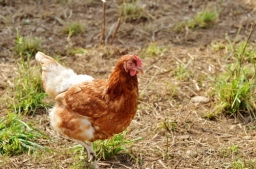# Weighs 81511

2 hens weigh 1kg more than a goose.
3 hens weigh 1kg more than 2 geese.
How much do one hen and one goose weigh?
When every goose weighs the same, and every hen weighs the same.

s =  1 kg
h =  1 kg

### Step-by-step explanation:Did you find an error or inaccuracy? Feel free to write us. Thank you!

Tips for related online calculators
Do you have a linear equation or system of equations and looking for its solution? Or do you have a quadratic equation?